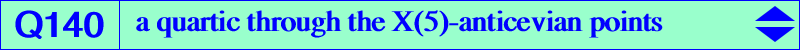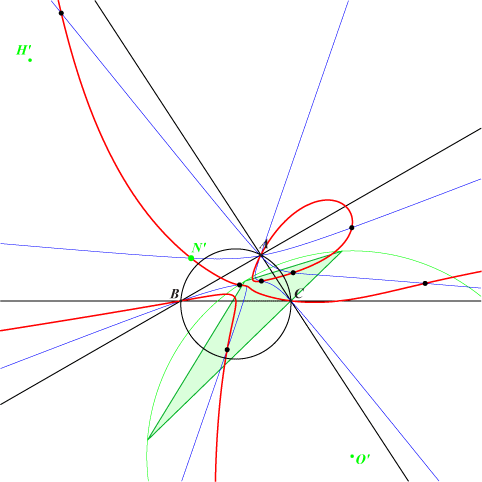too complicated to be written here. Click on the link to download a text file.X(1), X(2), X(3), X(15), X(16), X(17), X(18), X(39), X(2896), X(3413), X(3414) midpoints of ABC excenters points of K655 on (O)Q140 is a circular quartic passing through the X(5)-anticevian points. See Table 28. There are 10 (not always all real) such points. They are represented in black and green on the figure. For example, the nine-point center of the anticevian triangle of N' is N' itself. O' and H' are the corresponding circumcenter and orthocenter. *** The singular focus of Q104 is X(2080). Q140 has two real asymptotes parallel to those of the Kiepert hyperbola at X = 2a^4+b^4+c^4+4 b^2 c^2-4 a^2 b^2-4 a^2 c^2 : : , with SEARCH = 8.11132214901875. X lies on the lines {99,754}, {194,6680}, {230,538}, {325,543}, etc. X is now X(14148) in ETC (2017-08-21).The remaining points on the sidelines of ABC are {0 : -a^2+2 b^2+c^2 : a^2-b^2-2 c^2}, {2 a^2-b^2+c^2 : 0 : -a^2+b^2-2 c^2}, {2 a^2+b^2-c^2 : -a^2-2 b^2+c^2 : 0}.